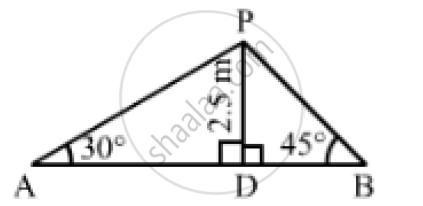# From a Point on a Bridge Across a River, Angles of Depression of the Banks on Opposite Sides of River Are 30° and 45° Respectively. If the Bridge is at a Height of 2.5m ,Find the Width of the River. - Mathematics

From a point on a bridge across a river, the angles of depression of the banks on opposite sides of the river are 30° and 45° respectively. If the bridge is at a height of 2.5m from the banks, find the width of the river.

#### SolutionLet A and B be two points on the banks on the opposite side of the river and P be the point on the bridge at a height of 2.5 m.

Thus, we have:
DP = 2.5, ∠PAD = 30°  and ∠PBD = 45°
In the right  ΔAPD,we have:

(DP)/(AD) = tan 30° = 1/sqrt(3)

⇒ 2.5 /(AD) = 1/ sqrt(3)

⇒ AD = 2.5 sqrt(3) m

In the right   ΔPDB,we have:

(DP)/(BD) = tan 45° = 1

⇒ 2.5/(BD) = 1

⇒ BD=2.5m

∴"Width of the river" = AB = ( AD+ BD) =(2.5sqrt(3) + 2.5) = 6.83m

Concept: Heights and Distances
Is there an error in this question or solution?
Chapter 14: Height and Distance - Exercises

#### APPEARS IN

RS Aggarwal Secondary School Class 10 Maths
Chapter 14 Height and Distance
Exercises | Q 28

Share